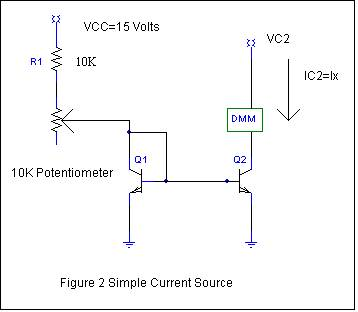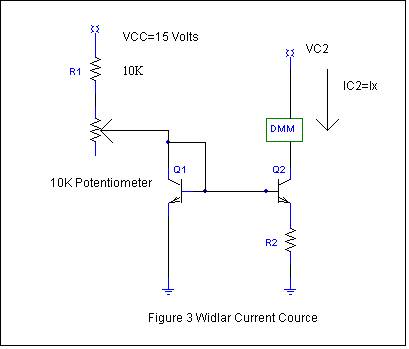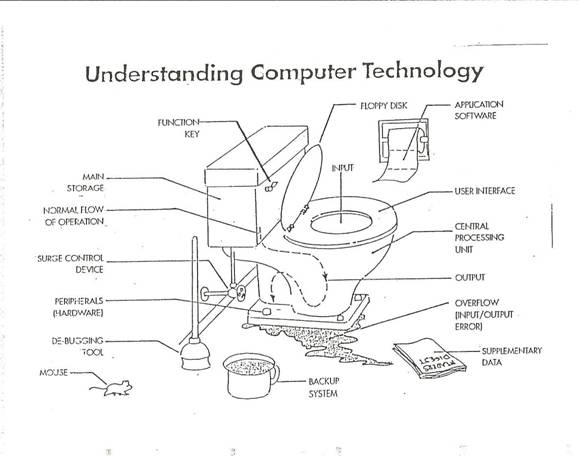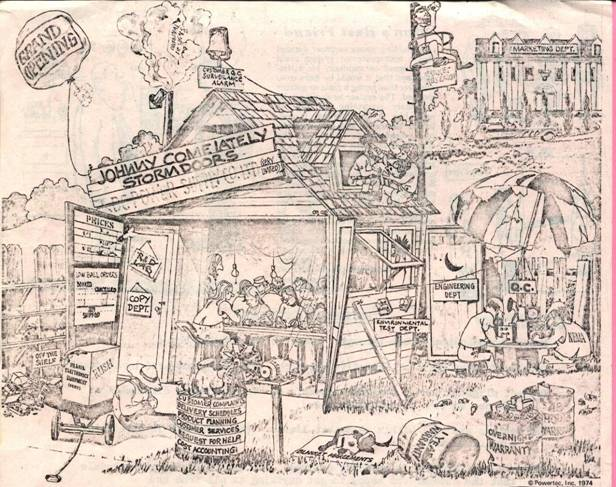EE 2212

EXPERIMENT 7

18 and 25 April

BJT CURRENT SOURCES AND THE EMITTER-COUPLED PAIR

Note 1:  Report is due Thursday, 2 May.  40 Points and 6 pages maximum not counting the cover sheet.

Note 2:  The CA 3046 is the same electrically as the LM 3046.  Just a different manufacturer.

PURPOSE

The purpose of this experiment is to characterize the  properties of a:

Ø Basic/Simple Current Source

Ø Widlar Current Source

Ø The emitter-coupled pair (DC transfer characteristics and AC gain measurements).

COMPONENTS

Ø LM3046/CA3046 transistor array.  The data sheet is posted on the class WEB page

Ø Resistors and potentiometers as required for the current sources.

Ø Three 20 kW resistors for the collector resistors of which two  should be reasonably well matched

Ø 4.7 kW resistor for the input voltage divider

Ø 47 W resistor for the input voltage divider

PRELAB FOR THE CURRENT SOURCES

Compute the values of the resistors you will need to evaluate the simple and Widlar current sources at the indicated current levels.

GENERAL INFORMATION

Ø    In IC biasing networks, it is essential that transistors be well matched and parameter variations track with temperature.   Figure 1 is a pin out of the LM3046/CA3046 Transistor Array. Observe that you MUST connect Pin 13, the IC substrate,  to the most negative point in the circuit or bad things happen to the IC.

Ø    The only reason there is a fixed 10 kW resistor in the circuit is to protect the BJT against inadvertent application of  a high voltage across the Base-Emitter junction as you adjust the potentiometer.  You do not want to apply 15 volts to the base of Q1 because the chip becomes toast (literally and figuratively)!!!  Effectively, the series combination of the 10 kW resistor and the potentiometer is the RREF.Figure 1 LM3046/CA3046 NPN BJT ARRAY

SIMPLE CURRENT SOURCE

Figure 2 is a schematic diagram of a simple current source.Connect the collector of Q2, (VC2) to a 5-volt DC supply. Place a DMM in series with the Q2 collector lead to measure current. Set IC2=IX to 1 mA.  Compare this value to the reference current.  Measure all key currents and voltages. Construct the I-V output characteristic by changing VC2 from 0 to 5 volts.   Obtain the output resistance from the slope. Compare to a SPICE simulation.

WIDLAR CURRENT SOURCE

Figure 3 is a schematic diagram of a Widlar current source.For a reference current of 1 mA, compute the value of R2 required to obtain Ix = 100 mA ±10%. Note that VCC = 15 volts. Now connect the collector of Q2 (VC2) to a 5-volt DC supply. Place a DMM in series with the Q2 collector lead to measure current. You may have to change the value of R2 from the computed value  to come within 100 mA ±10% .  Measure all key currents and voltages. Sketch  the I-V output characteristic from VC2 from 0 to 5 volts.. Compare these results with the simple current source results.  You will have to measure carefully because the slope will be close to flat as you would expect.  Compare to a SPICE simulation.

This is a good place to stop after Week 1.  You may also take the opportunity to finish the BJT experiment cut short by the closing of UMD.

……………………………………………………………………………………………………………………………….

PRELAB FOR THE EMITTER-COUPLED PAIR

Use Figure 4  and class notes for guidance to prepare a detailed circuit diagram.   Include  pinouts for  the LM3046/CA3046 npn array. From your circuit diagram and circuit specifications, calculate the expected important  Q-point values,  and Adm, Acm, and the CMRR in dB.

DC MEASUREMENTS

Refer to the diagram and data sheet of the LM 3046/CA3046 BJT array.

Set up the circuit in Figure 4  using Q1 and Q2 for the emitter-coupled pair. Q3 and Q4 form a simple current source.  Ground both the inputs of Q1 and Q2. Measure the all Q-point voltages and currents using the DMM.  Use the oscilloscope to also check for excessive noise which may translate as a noisy dc voltage measurement.   Pay particular attention to VOD. Since the transistors and resistors are reasonably well matched, you would expect VOD = 0 or reasonably close. If VOD is larger than a few tens of mV, check your circuit and/or match the collector resistors better.  Lead dress and length is also important.  Be neat!  Compare your Q-point values with the expected and PSPICE simulations.  In addition to using the DMM, look for excessive noise using the scope even though you are measuring the dc voltage matching.Figure 4

TRANSFER CHARACTERISTICS

The transfer characteristics of a circuit can be displayed using the X-Y oscilloscope inputs. The amplitude of the input must be large enough to drive the input through the entire desired range of operation. You are particularly interested in the VOD versus VID characteristic. Use a low frequency sinusoid or triangular wave as the input. From a practical viewpoint, if the input signals are noisy because of low amplitudes, you may choose to use an input voltage divider to provide "cleaner" waveforms. Consider implementing the 100:1 voltage divider input drive circuits, Figure 5,  although it doesn’t have to be 100:1.  The signal generators have a 100 mV minimum.  By using a 100:1 external divider, you can achieve a relatively noise free signal at the input to the BJT bases.  Keep track of the divider ratio you finally use to scale your measurement correctly. Also observe that because the oscilloscope does not have a floating input (i.e., one side of each oscilloscope input is connected to ground), you will have to measure either VO1 or VO2 and scale the final results accordingly by a factor of 2 and also do not forget the sign (180°phase) differences for each of the outputs.

Show that the slope of the transfer characteristic will be equal to |Adm/2|. Compare your results to a SPICE simulation.Figure 5

DIFFERENTIAL-MODE OPERATION

Set up your input signals, use 1 kHz, so that the output is reasonably linear. You will need some level of voltage division as shown in the figure.   The figure illustrates a 100:1 divider but the actual divider value is not critical.  Use the oscilloscope and DMM to measure the differential-mode voltage gain. Compare your results to your calculations and a SPICE simulation.  Include  the effect of a non-infinite Early voltage to improve your analysis and simulation accuracy.

If you  decide to pursue a BSEE degree, you should at least understand the basics of computer engineering.   Above and beyond CS1, the following provides an important understanding of computer technology hardware.And for those of you with an internship this summer should be aware of the corporate hieraccy.: